# Python滤镜和图像风格迁移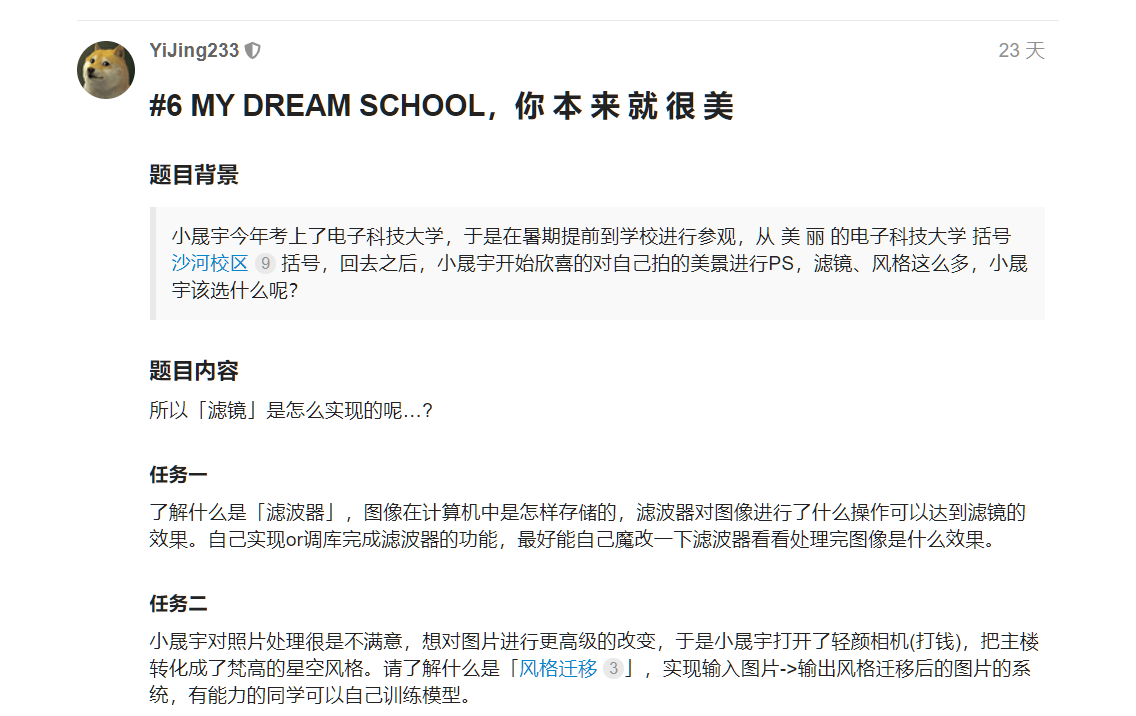## 任务一

### 1.了解滤波器

#### 一、图像本质上就是各种色彩波的叠加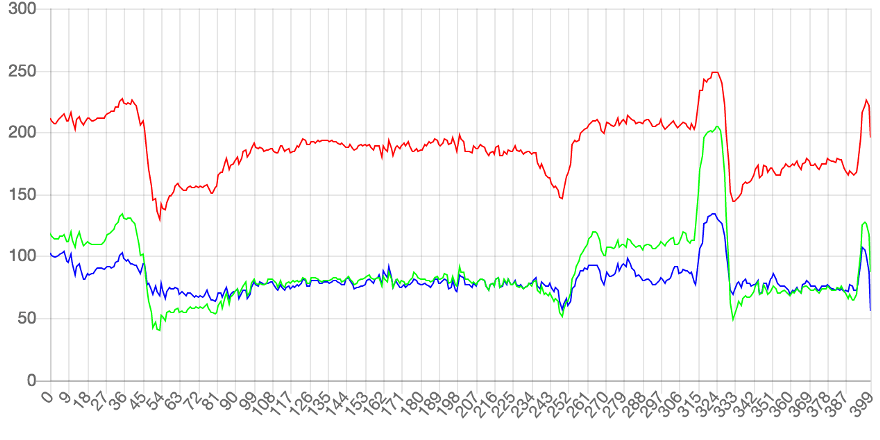• 低通滤波器（lowpass）：减弱或阻隔高频信号，保留低频信号

• 高通滤波器（highpass）：减弱或阻隔低频信号，保留高频信号

• 低通滤波器：图像变得模糊（锐度下降）• 高通滤波器：图像只剩下锐度极高的部分，其他部分的色彩丢失虽然实际应用的滤镜比单纯的高通低通滤波器复杂，但本质上应该也是附加规则的高低通滤波器的组合。

#### 二、理解卷积算法

PIL库中的滤镜算法主要涉及到卷积滤镜，即在数字图像的像素矩阵中使用一个n*n的矩阵来滤波(该矩阵即卷积核kernal)，以这个矩阵为单位对图像像素进行遍历，每个输出的像素都是区域像素按照一定权重组合计算出的结果，遍历之后输出的图像就是输出的图像。(即依据“规则”通过每个像素点附近的像素值来修改当前像素点的值，遍历修改后就完成了滤波)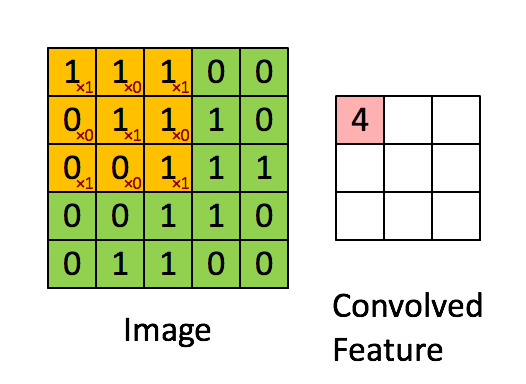这张Gif很好的描述了卷积算法的过程，所以被我偷了下来，嘻嘻

### 2.尝试使用现成的滤波器

• BLUR：模糊滤波

• CONTOUR：轮廓滤波

• DETAIL：细节滤波

• EDGE_ENHANCE：边界增强滤波

• EDGE_ENHANCE_MORE：边界增强滤波（程度更深）

• EMBOSS：浮雕滤波

• FIND_EDGES：寻找边界滤波

• SMOOTH：平滑滤波

• SMOOTH_MORE：平滑滤波（程度更深）

• SHARPEN：锐化滤波

​ >percent指定反锐化强度（百分比）;

​ >threshold控制被锐化的最小亮度变化。

• Kernel(size, kernel, scale=None, offset=0)：核滤波

​ 当前版本只支持核大小为3x3和5x5的核大小，且图像格式为“L”和“RGB”的图像。

​ >size指定核大小（width, height）；

​ >kernel指定核权值的序列；

​ >scale指定缩放因子；

​ >offset指定偏移量，如果使用，则将该值加到缩放后的结果上。

• RankFilter(size, rank)：排序滤波

​ >size指定滤波核的大小；

​ >rank指定选取排在第rank位的像素，若大小为0，则为最小值滤波；若大小为size * size / 2则为中值滤波；若大小为size * size - 1则为最大值滤波。

• MedianFilter(size=3)：中值滤波

​ >size指定核的大小

• MinFilter(size=3)：最小值滤波器

​ >size指定核的大小

MaxFilter(size=3)：最大值滤波器

​ >size指定核的大小

ModeFilter(size=3)**：波形滤波器

​ 选取核内出现频次最高的像素值作为该点像素值，仅出现一次或两次的像素将被忽略，若没有像素出现两次以上，则保留原像素值。

​ >size指定核的大小

 1 2 3 4 5 6 7 8 9  from PIL import ImageFilter from PIL import Image im = Image.open("test.png") im_blur = im.filter(ImageFilter.GaussianBlur(radius=5)) # *高斯模糊 im_contour = im.filter(ImageFilter.CONTOUR) # *轮廓滤波 im.show() im_blur.show() im_contour.show()### 3.自己实现卷积滤波器

#### 1.伟大的第一步，确定算法基本思路

1. 初始化设置卷积核
2. 读取图像二维列表形式存储的像素值
3. 执行遍历获取新的像素值并存储到新的列表中

#### 2.尝试实现

 1 2 3  core = np.array([[1, 1, 0], [1, 1, -1], [0, -1, -1]]) # *预设值的卷积核 

 1 2 3 4 5 6 7 8  img = plt.imread("t1.jpg") core_line = core.shape // 2 # *获取卷积核行数 core_row = core.shape // 2 # *获取卷积核列数 # *分别在行前后添加i行,在列前后添加j列,第三维不填充,填充值为0(不会影响原图像) img = np.pad(img, ((core_line, core_line), (core_row, core_row), (0, 0)), 'constant') channel_r = img[:, :, 0] # *R通道像素值 channel_g = img[:, :, 1] # *G通道像素值 channel_b = img[:, :, 2] # *B通道像素值 

  1 2 3 4 5 6 7 8 9 10 11 12 13 14 15 16 17 18  def calculate(img, core): result = (img * core).sum() # *矩阵乘法获得结果像素值 if(result < 0): # *过滤无效像素值 result = 0 elif result > 255: result = 255 return result channel_line = img.shape - core_line + 1 # *获取图像像素点列数 channel_row = img.shape - core_row + 1 # *获取图像像素点行数 new_img = np.zeros((channel_line, channel_row), dtype='uint8') # *初始化一个和原图像大小相同的用0填充的图像矩阵 for i in trange(channel_line): for j in range(channel_row): # *调用calculate函数完成每个像素点的滤波计算并赋值给新图像 new_img[i][j] = calculate(img[i:i+core_line, j:j+core_row], core) 

  1 2 3 4 5 6 7 8 9 10 11 12 13 14 15 16 17 18 19 20 21 22 23 24 25 26 27 28 29 30 31 32 33 34 35 36 37 38 39 40 41 42 43 44 45 46 47 48 49 50 51 52 53 54 55 56 57  import matplotlib.pyplot as plt import pylab import numpy as np from tqdm import trange def collect_channel(img, core): core_line = core.shape // 2 # *获取卷积核行数 core_row = core.shape // 2 # *获取卷积核列数 # *分别在行前后添加i行,在列前后添加j列,第三维不填充,填充值为0(不会影响原图像) img = np.pad(img, ((core_line, core_line), (core_row, core_row), (0, 0)), 'constant') channel_r = convolution(img[:, :, 0], core) # *提取R通道数据并执行卷积 channel_g = convolution(img[:, :, 1], core) # *提取G通道数据并执行卷积 channel_b = convolution(img[:, :, 2], core) # *提取B通道数据并执行卷积 dstack = np.dstack([channel_r, channel_g, channel_b]) return dstack # *合并三个颜色通道 def convolution(img, core): core_line = core.shape # *获取卷积核行数 core_row = core.shape # *获取卷积核列数 channel_line = img.shape - core_line + 1 # *获取图像像素点列数 channel_row = img.shape - core_row + 1 # *获取图像像素点行数 new_img = np.zeros((channel_line, channel_row), dtype='uint8') # *初始化一个和原图像大小相同的用0填充的图像矩阵 for i in trange(channel_line): for j in range(channel_row): # *调用calculate函数完成每个像素点的滤波计算并赋值给新图像 new_img[i][j] = calculate(img[i:i+core_line, j:j+core_row], core) return new_img def calculate(img, core): result = (img * core).sum() # *矩阵乘法获得结果像素值 if(result < 0): # *过滤无效像素值 result = 0 elif result > 255: result = 255 return result img = plt.imread("t1.jpg") core = np.array([[1, 1, 0], [1, 1, -1], [0, -1, -1]]) # *预设的卷积核 result = collect_channel(img, core) plt.imshow(result) plt.imsave("D:/0aJotang/#6/results.jpg", result) pylab.show()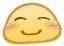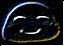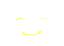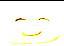#### 3.均值模糊/高斯模糊

• 均值模糊

​ 直接在卷积遍历过程中求整个矩阵的平均值并赋值给对应像素点：

修改求和函数即可做到

效果如图:

 1 2 3 4 5 6 7  def calculate(img, core): result = (img * core).sum() / 9 # *矩阵乘法获得结果像素值并求平均值 if(result < 0): # *过滤无效像素值 result = 0 elif result > 255: result = 255 return result 

效果举例：• 高斯模糊

​ 因为图像像素点分布实际上不是简单分布，每个像素点附近的像素点存在一定的连续性，距离越远，连续性就越不明显，这样的分布特点和正态分布一致，于是有使用正态分布(高斯函数)的方式来模糊处理图像的算法，这样的模糊方法因为过渡更加符合现实情况，在实拍的图片中使用效果会显得更加真实。

​ 因为是二维的图像，所以需要使用到二维高斯函数：

$$G(x,y)=\frac1{2\mathrm{πσ}^2}e^\frac{ { }^{-(x^2+y^2)} } {2\sigma^2}$$

​ 其中，σ为模糊量，因为在正态分布中σ为方差，其值越大曲线越扁平，相应的模糊过渡越平滑，所以模糊量越大

​ 此外，卷积核的半径与模糊程度也呈现正相关关系

  1 2 3 4 5 6 7 8 9 10 11 12 13 14 15 16 17 18 19 20 21 22 23 24 25 26 27 28 29 30 31 32 33 34 35 36 37 38 39 40 41 42 43 44 45 46 47 48 49 50 51 52 53 54 55 56 57 58 59 60 61 62 63 64 65 66 67 68 69 70 71 72 73 74 75 76 77 78 79 80 81  import matplotlib.pyplot as plt from matplotlib.pyplot import pause import pylab import numpy as np from tqdm import trange # *设置全局变量 def public(): global core_line, core_row, sigma core_line = 10 core_row = 10 sigma = 5 # *计算二维高斯函数值 def gaussian(sigma, x, y): z = 1/(2 * np.pi * (sigma**2)) * np.exp(-(x**2+y**2)/(2 * sigma**2)) return z def collect_channel(img, core): public() # *分别在行前后添加i行,在列前后添加j列,第三维不填充,填充值为0(不会影响原图像) img = np.pad(img, ((core_line, core_line), (core_row, core_row), (0, 0)), 'constant') channel_r = convolution(img[:, :, 0], core) # *提取R通道数据并执行卷积 channel_g = convolution(img[:, :, 1], core) # *提取G通道数据并执行卷积 channel_b = convolution(img[:, :, 2], core) # *提取B通道数据并执行卷积 dstack = np.dstack([channel_r, channel_g, channel_b]) return dstack # *合并三个颜色通道 def convolution(img, core): public() channel_line = img.shape - core_line + 1 # *获取图像像素点列数 channel_row = img.shape - core_row + 1 # *获取图像像素点行数 new_img = np.zeros((channel_line, channel_row), dtype='uint8') # *初始化一个和原图像大小相同的用0填充的图像矩阵 for i in trange(channel_line): for j in range(channel_row): # *调用calculate函数完成每个像素点的滤波计算并赋值给新图像 new_img[i][j] = calculate(img[i:i+core_line, j:j+core_row], core) return new_img def calculate(img, core): result = (img * core).sum() # *矩阵乘法获得结果像素值 if(result < 0): # *过滤无效像素值 result = 0 elif result > 255: result = 255 return result def gaussian_cal(): public() # *初始化卷积核 core = [[1.0 for i in range(core_line)] for j in range(core_row)] sums = 0 # *计算卷积核半径 for i in range(core_line): for j in range(core_row): x = abs(j - (core_row // 2)) y = abs(i - (core_line // 2)) core[i][j] = gaussian(sigma, x, y) sums = sums + core[i][j] core = core / sums # *保证卷积核元素总和为1 return core img = plt.imread("D:/0aJotang/#6/nice.jpg") plt.imshow(img) result = collect_channel(img, gaussian_cal()) plt.imshow(result) plt.imsave("D:/0aJotang/#6/results1.jpg", result) pylab.show()#### 4.其他滤镜

• 浮雕滤镜$$\begin{array} {ccc}-1&0&0\0&1&0\0&0&0\end{array}$$

• 轮廓提取$$\begin{array} {ccc}-1&-1&-1\-1&8&-1\-1&-1&-1\end{array}$$

• 更多滤镜，魔改卷积核······

## 任务二

### 1.认识图片风格迁移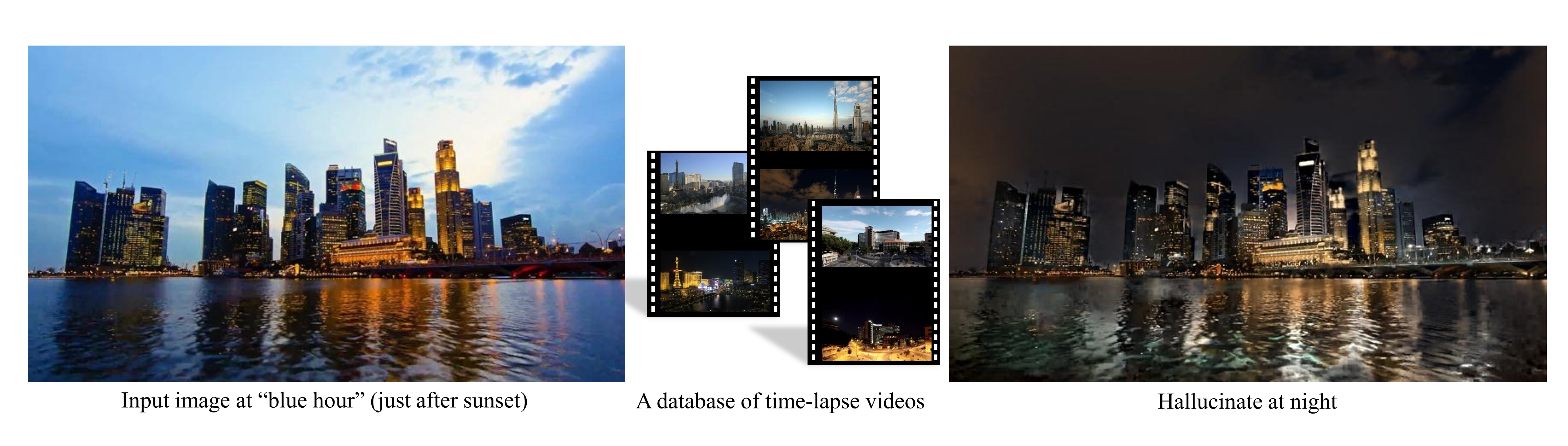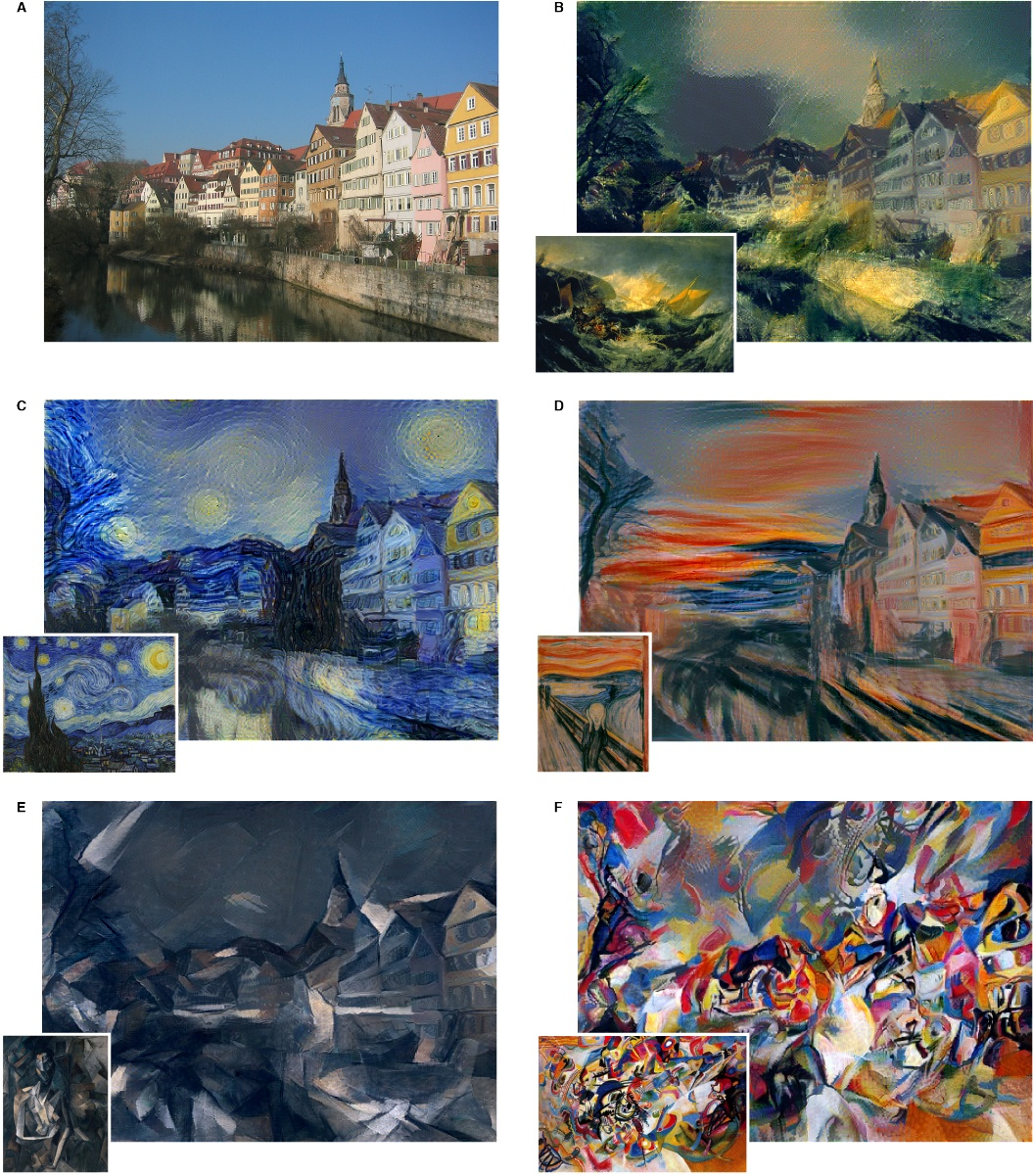### 2.使用pytorch实现图片风格迁移

#### 1.核心思想

• 计算风格损失和内容损失，并逐步降低梯度优化损失，最后优化参数输出
• 通过预训练的卷积网络提取出更高纬度的图片内容和风格，最后通过定义内容损失函数和风格损失函数进行反向传播更新参数

#### 2.功能分步实现

##### 1.加载图片
  1 2 3 4 5 6 7 8 9 10 11 12 13 14 15 16 17 18 19 20 21 22 23 24  # *输出图像大小 imsize = 512 loader = transforms.Compose([ transforms.Resize(imsize), # *拉伸调整输入图像尺寸 transforms.ToTensor()]) # *将图像转换为torch张量 # *图像载入 def image_loader(image_name): image = Image.open(image_name) image = loader(image).unsqueeze(0) # *添加伪维数以满足神经网络要求的输入维数 return image.to(device, torch.float) # *转换并绘制图像 def imshow(tensor, title=None): unloader = transforms.ToPILImage() image = tensor.cpu().clone() # *clone张量 image = image.squeeze(0) # *移除添加的伪维 image = unloader(image) # *转换回PIL图像 plt.imshow(image) # *绘制图像 if title is not None: plt.title(title) plt.pause(0.001) # *暂停使plot子图更新 
##### 2.计算损失
1. 内容损失 $$L_{content}\left(\overset\rightharpoonup p,\overset\rightharpoonup x,l\right)=\frac12\sum_{i,j} {(F_{i,j}^l-P_{i,j}^l)}^2\$$

  1 2 3 4 5 6 7 8 9 10 11  # *内容损失 class ContentLoss(nn.Module): def __init__(self, target,): super(ContentLoss, self).__init__() self.target = target.detach() def forward(self, input): self.loss = F.mse_loss(input, self.target) # *调用mse_loss计算矩阵均方损失 return input 
2. 风格损失

style loss取自原始image和生成的image在神经网络中的Gram matrix的MSE(Gram矩阵可以在一定程度上反映原始图像的“风格”):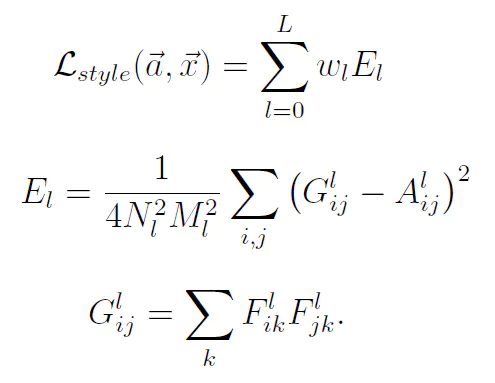因为公式字母太多写LaTex太麻烦于是就用了图片XD

  1 2 3 4 5 6 7 8 9 10 11 12 13 14 15 16 17 18 19 20 21 22 23 24 25 26  # *计算Gram矩阵 def gram_matrix(input): a, b, c, d = input.size() # a=batch size(=1) # *特征映射 b=number # *(c,d)=dimensions of a f. map (N=c*d) features = input.view(a * b, c * d) # *将矩阵F_XL重塑为\hat F_XL G = torch.mm(features, features.t()) # *计算gram积 # *归一化gram矩阵的值. return G.div(a * b * c * d) # *风格损失计算(与内容损失计算类似) class StyleLoss(nn.Module): def __init__(self, target_feature): super(StyleLoss, self).__init__() self.target = gram_matrix(target_feature).detach() def forward(self, input): G = gram_matrix(input) self.loss = F.mse_loss(G, self.target) return input 
##### 3.降低梯度
 1 2 3   def get_input_optimizer(input_img): optimizer = optim.LBFGS([input_img.requires_grad_()]) return optimizer 
##### 4.规范化输入图像以导入nn.Sequential
  1 2 3 4 5 6 7 8 9 10  class Normalization(nn.Module): def __init__(self, mean, std): super(Normalization, self).__init__() # *计算均值、均方差以归一化矩阵 self.mean = torch.tensor(mean).view(-1, 1, 1) self.std = torch.tensor(std).view(-1, 1, 1) def forward(self, img): # *归一化矩阵 return (img - self.mean) / self.std 
##### 5.获得风格模型和损失量
  1 2 3 4 5 6 7 8 9 10 11 12 13 14 15 16 17 18 19 20 21 22 23 24 25 26 27 28 29 30 31 32 33 34 35 36 37 38 39 40 41 42 43 44 45 46 47 48 49 50 51 52 53 54 55 56 57 58 59 60  # *获得风格模型和损失量 def get_style_model_and_losses(cnn, normalization_mean, normalization_std, style_img, content_img, content_layers=content_layers_default, style_layers=style_layers_default): cnn = copy.deepcopy(cnn) # *规范化模块 normalization = Normalization( normalization_mean, normalization_std).to(device) # *只是为了拥有可迭代的访问权限或列出内容/系统损失 content_losses = [] style_losses = [] # *创建一个新的nn.Sequential来放入按序激活的模块 model = nn.Sequential(normalization) i = 0 # *每次检视层的增量 for layer in cnn.children(): if isinstance(layer, nn.Conv2d): i += 1 name = 'conv_{}'.format(i) elif isinstance(layer, nn.ReLU): name = 'relu_{}'.format(i) # *在下面插入的ContentLoss和StyleLoss的本地版本不能很好地发挥作用。所以在这里替换不合适的 layer = nn.ReLU(inplace=False) elif isinstance(layer, nn.MaxPool2d): name = 'pool_{}'.format(i) elif isinstance(layer, nn.BatchNorm2d): name = 'bn_{}'.format(i) else: raise RuntimeError('Unrecognized layer: {}'.format( layer.__class__.__name__)) model.add_module(name, layer) if name in content_layers: # *加入内容损失: target = model(content_img).detach() content_loss = ContentLoss(target) model.add_module("content_loss_{}".format(i), content_loss) content_losses.append(content_loss) if name in style_layers: # *加入风格损失: target_feature = model(style_img).detach() style_loss = StyleLoss(target_feature) model.add_module("style_loss_{}".format(i), style_loss) style_losses.append(style_loss) # *移除添加的损失层 for i in range(len(model) - 1, -1, -1): if isinstance(model[i], ContentLoss) or isinstance(model[i], StyleLoss): break model = model[:(i + 1)] return model, style_losses, content_losses 

#### 3.完整示例代码

  1 2 3 4 5 6 7 8 9 10 11 12 13 14 15 16 17 18 19 20 21 22 23 24 25 26 27 28 29 30 31 32 33 34 35 36 37 38 39 40 41 42 43 44 45 46 47 48 49 50 51 52 53 54 55 56 57 58 59 60 61 62 63 64 65 66 67 68 69 70 71 72 73 74 75 76 77 78 79 80 81 82 83 84 85 86 87 88 89 90 91 92 93 94 95 96 97 98 99 100 101 102 103 104 105 106 107 108 109 110 111 112 113 114 115 116 117 118 119 120 121 122 123 124 125 126 127 128 129 130 131 132 133 134 135 136 137 138 139 140 141 142 143 144 145 146 147 148 149 150 151 152 153 154 155 156 157 158 159 160 161 162 163 164 165 166 167 168 169 170 171 172 173 174 175 176 177 178 179 180 181 182 183 184 185 186 187 188 189 190 191 192 193 194 195 196 197 198 199 200 201 202 203 204 205 206 207 208 209 210 211 212 213 214 215 216 217 218 219 220 221 222 223 224 225 226 227 228 229 230 231 232 233 234 235 236 237 238 239 240 241 242 243 244 245 246 247 248 249 250 251 252 253  from __future__ import print_function import torch import torch.nn as nn import torch.nn.functional as F import torch.optim as optim from PIL import Image import matplotlib.pyplot as plt import torchvision.transforms as transforms import torchvision.models as models import copy # *指定计算设备 device = torch.device("cuda" if torch.cuda.is_available() else "cpu") # *输出图像大小 imsize = 512 loader = transforms.Compose([ transforms.Resize(imsize), # *拉伸调整输入图像尺寸 transforms.ToTensor()]) # *将图像转换为torch张量 # *图像载入 def image_loader(image_name): image = Image.open(image_name) # *添加伪维数以满足神经网络要求的输入维数 image = loader(image).unsqueeze(0) return image.to(device, torch.float) style_img = image_loader("D:/0aJotang/#6/py_fi/input_style/style.jpg") # *读取风格图像 content_img = image_loader("D:/0aJotang/#6/py_fi/input_content/content.jpg") # *读取内容图像 # *确认传入内容和风格图像尺寸一致 assert style_img.size() == content_img.size(), \ "we need to import style and content images of the same size" unloader = transforms.ToPILImage() # *将图像转换回PIL—image以在plot绘制 plt.ion() # *用于转换并绘制图像 def imshow(tensor, title=None): image = tensor.cpu().clone() # *clone张量 image = image.squeeze(0) # *移除添加的伪维 image = unloader(image) # *重新转换回PIL图像 plt.imshow(image) # *绘制图像 if title is not None: plt.title(title) plt.pause(0.001) # *暂停使plot子图更新 # *内容损失 class ContentLoss(nn.Module): def __init__(self, target,): super(ContentLoss, self).__init__() self.target = target.detach() def forward(self, input): self.loss = F.mse_loss(input, self.target) # *计算损失 return input # *计算gram矩阵 def gram_matrix(input): a, b, c, d = input.size() # a=batch size(=1) # *特征映射 b=number # *(c,d)=dimensions of a f. map (N=c*d) features = input.view(a * b, c * d) # *将矩阵F_XL重塑为\hat F_XL G = torch.mm(features, features.t()) # *计算gram积 # *归一化gram矩阵的值. return G.div(a * b * c * d) # *风格损失计算 class StyleLoss(nn.Module): def __init__(self, target_feature): super(StyleLoss, self).__init__() self.target = gram_matrix(target_feature).detach() def forward(self, input): G = gram_matrix(input) self.loss = F.mse_loss(G, self.target) return input # *导入预训练模型 cnn = models.vgg19(pretrained=True).features.to(device).eval() cnn_normalization_mean = torch.tensor([0.485, 0.456, 0.406]).to(device) cnn_normalization_std = torch.tensor([0.229, 0.224, 0.225]).to(device) # *规范化输入图像以导入nn.Sequential class Normalization(nn.Module): def __init__(self, mean, std): super(Normalization, self).__init__() # *计算均值、均方差以归一化矩阵 self.mean = torch.tensor(mean).view(-1, 1, 1) self.std = torch.tensor(std).view(-1, 1, 1) def forward(self, img): # *归一化矩阵 return (img - self.mean) / self.std # *计算样式/内容损失的期望深度层： content_layers_default = ['conv_4'] style_layers_default = ['conv_1', 'conv_2', 'conv_3', 'conv_4', 'conv_5'] # *获得风格模型和损失量 def get_style_model_and_losses(cnn, normalization_mean, normalization_std, style_img, content_img, content_layers=content_layers_default, style_layers=style_layers_default): cnn = copy.deepcopy(cnn) # *规范化模块 normalization = Normalization( normalization_mean, normalization_std).to(device) # *只是为了拥有可迭代的访问权限或列出内容/系统损失 content_losses = [] style_losses = [] # *创建一个新的nn.Sequential来放入按序激活的模块 model = nn.Sequential(normalization) i = 0 # *每次检视层的增量 for layer in cnn.children(): if isinstance(layer, nn.Conv2d): i += 1 name = 'conv_{}'.format(i) elif isinstance(layer, nn.ReLU): name = 'relu_{}'.format(i) # *对于我们在下面插入的ContentLoss和StyleLoss， # *本地版本不能很好地发挥作用。所以我们在这里替换不合适的 layer = nn.ReLU(inplace=False) elif isinstance(layer, nn.MaxPool2d): name = 'pool_{}'.format(i) elif isinstance(layer, nn.BatchNorm2d): name = 'bn_{}'.format(i) else: raise RuntimeError('Unrecognized layer: {}'.format( layer.__class__.__name__)) model.add_module(name, layer) if name in content_layers: # *加入内容损失: target = model(content_img).detach() content_loss = ContentLoss(target) model.add_module("content_loss_{}".format(i), content_loss) content_losses.append(content_loss) if name in style_layers: # *加入风格损失: target_feature = model(style_img).detach() style_loss = StyleLoss(target_feature) model.add_module("style_loss_{}".format(i), style_loss) style_losses.append(style_loss) # *移除添加的损失层 for i in range(len(model) - 1, -1, -1): if isinstance(model[i], ContentLoss) or isinstance(model[i], StyleLoss): break model = model[:(i + 1)] return model, style_losses, content_losses input_img = content_img.clone() # *读取并clone内容图片 # *降低梯度 def get_input_optimizer(input_img): optimizer = optim.LBFGS([input_img.requires_grad_()]) return optimizer # *运行风格迁移 def run_style_transfer(cnn, normalization_mean, normalization_std, content_img, style_img, input_img, num_steps=300, style_weight=1000000, content_weight=1): print('Building the style transfer model..') model, style_losses, content_losses = get_style_model_and_losses(cnn, normalization_mean, normalization_std, style_img, content_img) optimizer = get_input_optimizer(input_img) print('Optimizing..') run =  while run <= num_steps: def closure(): # *修正更新的输入图像的值 input_img.data.clamp_(0, 1) optimizer.zero_grad() model(input_img) style_score = 0 content_score = 0 for sl in style_losses: style_score += sl.loss for cl in content_losses: content_score += cl.loss style_score *= style_weight content_score *= content_weight loss = style_score + content_score loss.backward() run += 1 if run % 50 == 0: print("run {}:".format(run)) print('Style Loss : {:4f} Content Loss: {:4f}'.format( style_score.item(), content_score.item())) print() return style_score + content_score optimizer.step(closure) # *修正张量为(0,1) input_img.data.clamp_(0, 1) return input_img output = run_style_transfer(cnn, cnn_normalization_mean, cnn_normalization_std, content_img, style_img, input_img) plt.figure() imshow(output, title='Output Image') plt.savefig("D:/0aJotang/#6/py_fi/output/output.png") plt.ioff() plt.show()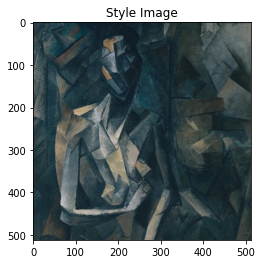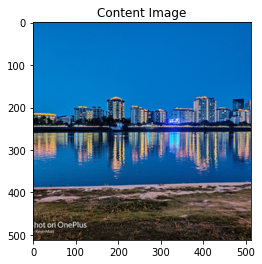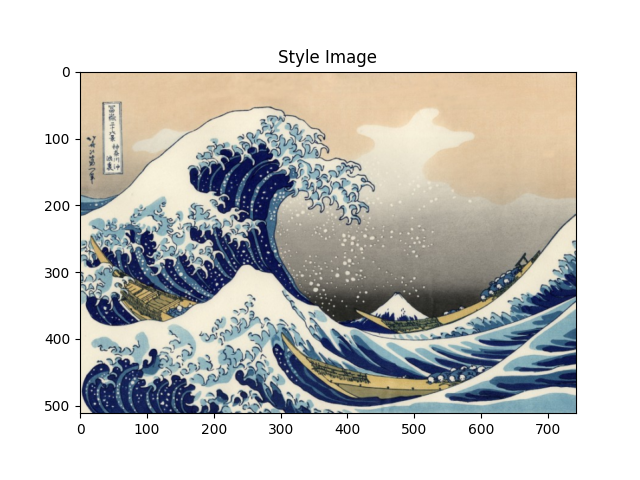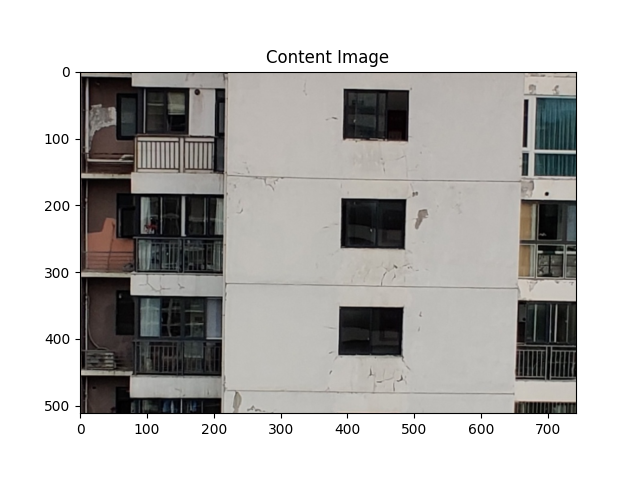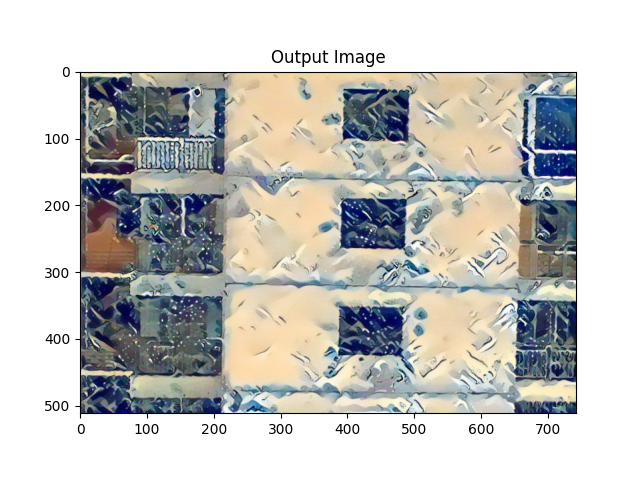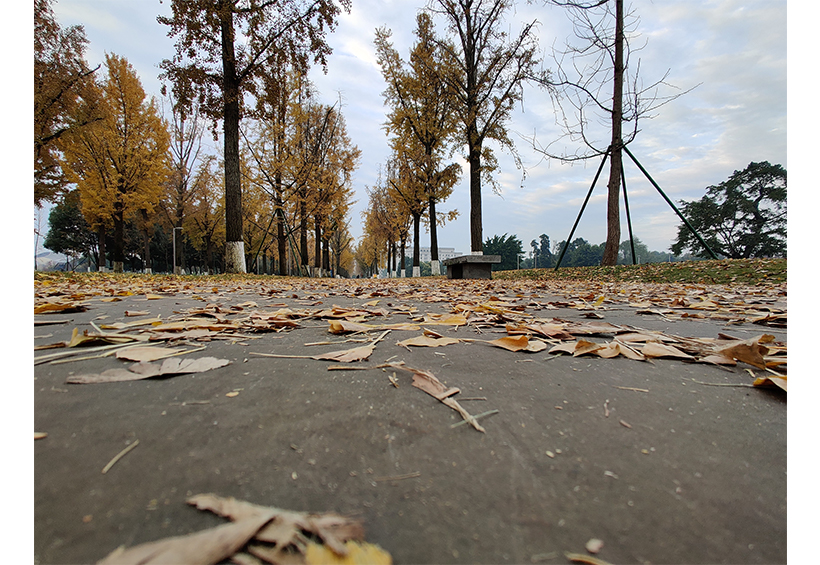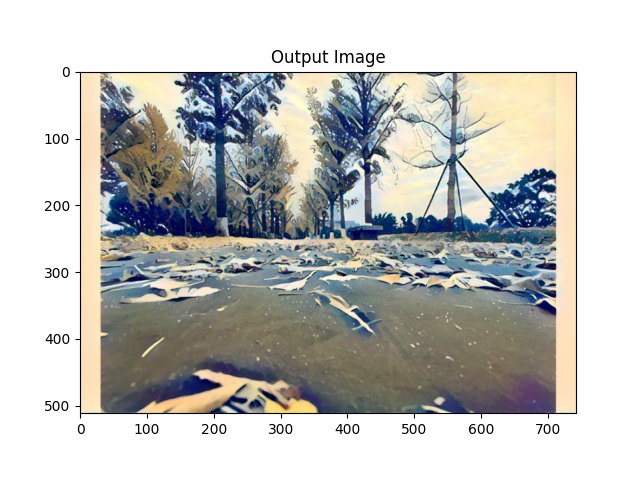da829fd
Update Blog